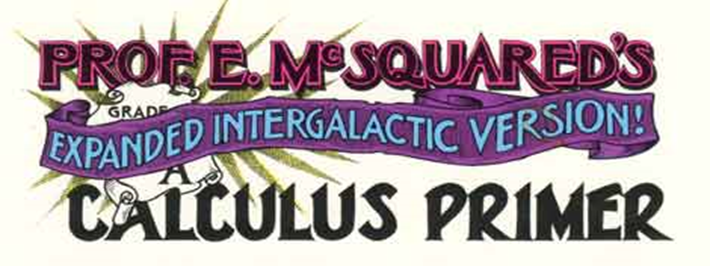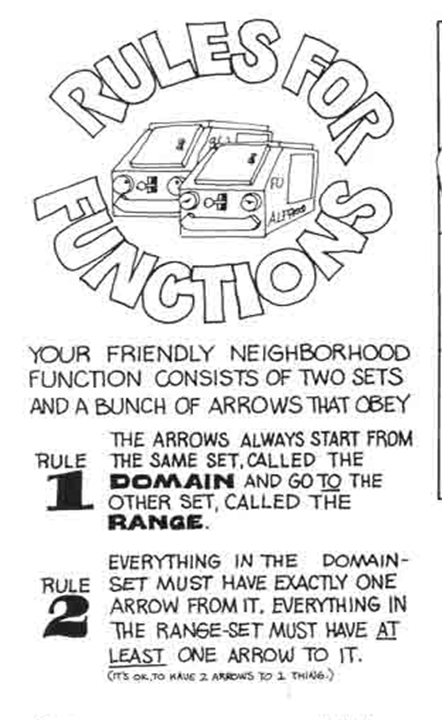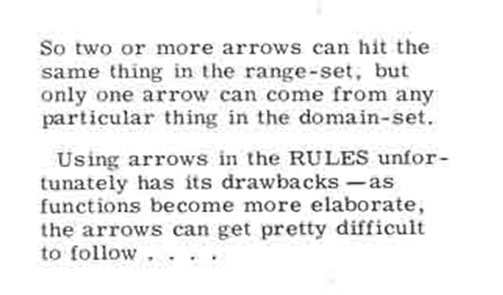Center for Recruitment and Retention of Mathematics Teachers
University of Arizona
¤
October 18, 2017

Using Mapping Diagrams to Make Sense of Functions and Calculus
Part I
Functions, Equations, Linearity
What was covered and the edge of Part II.

Martin Flashman
Professor of Mathematics
Humboldt State University

Abstract:
Participants will learn how to use mapping diagrams (MD) to make sense of functions and relate these to materials taught in calculus and in preparing for calculus.
A mapping diagram is an alternative to a Cartesian graph that visualizes a function using parallel axes. Like a table, it can present finite date, but also can be used dynamically with technology.
An overview of basic function concepts with MD’s will begin the session using worksheets and GeoGebra.
Connections of MD's to key concepts in studying calculus and preparing to study calculus will follow showing the power of MD’s to make sense of function concepts of measurement, rate, composition, and approximation related to calculus.

Background and examples will be available at Mapping Diagrams from A(lgebra) B(asics) to C(alculus) and D(ifferential) E(quation)s. A Reference and Resource for Function Visualizations Using Mapping Diagrams. http://flashman.neocities.org/MD/section-1.1VF.html

Outline for Workshop I  ¤
• Mapping Diagrams
• What is a Mapping Diagram?
• Functions: Tables, Mapping Diagrams, and Graphs
• Linear and Quadratic Functions and Equations
• An On-line Lesson on Linear Functions
• Solving equations visualized with mapping diagrams
• Composition
• Inverses
• Linear functions are the key to understanding calculus.
• Nonlinear ( 1/x, trig, exp/ln) Connect to Resource
• Limits and The Derivative (visualize rate/ratio)
• An On-line Lesson on the Derivative
• Limits with Mapping Diagrams
• The Derivative As A
• Number,
• Magnification
• Rate
• Vector
• The Chain Rule: Mapping Diagrams for Composite (Linear) Functions
• An On-line Lesson on the Chain Rule
• Continuity and Solving Equations
• The Intermediate Value Theorem
• Newton's Method.
• 1st and 2nd Derivative Analysis [Time permitting]
• Differentials, Differential Equations, and Euler's Method
• Mapping Diagrams for the Differential
• Differential Equations, Euler, Mapping Diagrams

1.Mapping Diagrams. ¤

1.1 What is a mapping diagram?
Introduction and simple examples from the past: Napiers LogarithmSee: http://www.maa.org/press/periodicals/convergence/logarithms-the-early-history-of-a-familiar-function-john-napier-introduces-logarithms

Logarithms: The Early History of a Familiar Function - John Napier Introduces Logarithms  by Kathleen M. Clark (The Florida State University) and Clemency Montelle (University of Canterbury)Written by Howard Swann and John Johnson
An early source for visualizing functions at an elementary level before calculus.